# MP Board Class 11th Maths Important Questions Chapter 9 Sequences and Series

## MP Board Class 11th Maths Important Questions Chapter 9 Sequences and Series Important Questions

### Sequences and Series Important Questions

Sequences and Series Objective Type Questions

(A) Choose the correct answer of the following:

Question 1.
The sum of the cube of first n positive integer is :
(a) $$\frac {n(n + 1)}{2}$$
(b) $$\frac {n(n + 1)(2n + 1)}{6}$$
(c) $$\frac {n(n + 1)(n + 2)}{6}$$
(d) {$$\frac {n(n + 1)}{2}$$}2
(d) {$$\frac {n(n + 1)}{2}$$}2

Make use of this free Radius of Convergence Calculator to get the radius of convergence of a power series. Online calculator tool gives output in no time.

Question 2.
The sum of n term of arithmetic progression is 2n + 3n2, its second term will be :
(a) 10
(b) 12
(c) 16
(d) 11
(c) 16Question 3.
If arithmetic mean of a and b is $$\frac { { a }^{ n }+{ n }^{ n } }{ { a }^{ n – 1 }+{ b }^{ n – 1 } }$$, then the value of n will be :
(a) 1
(b) 0
(c) – 1
(d) $$\frac {1}{2}$$
(a) 1

Question 4.
Which term of the series 8, 4,0, ………….. is – 24 :
(a) 7th
(b) 28th
(c) 8th
(d) 9th
(d) 9th

Question 5.
If the first term of arithmetic progression be a and last term is l, then the sum of n terms will be :
(a) $$\frac {n}{2}$$[2a – (n – 1)d]
(b) $$\frac {n}{2}$$[2a + (n – 1)d]
(c) $$\frac {n}{2}$$(a + l)
(d ) $$\frac {n}{2}$$(a – l).
(c) $$\frac {n}{2}$$(a + l)

Find the sequence calculator allows to calculate online the terms of the sequence whose index is between two limits.

Question 6.
The next term of the sequence 2$$\sqrt { 2 }$$, $$\sqrt { 2 }$$, 0, …………… is :
(a) – $$\sqrt { 3 }$$
(b) $$\frac { 1 }{ \sqrt { 2 } }$$
(c) – $$\sqrt { 2 }$$
(d) $$\sqrt { 2 }$$
(c) – $$\sqrt { 2 }$$

Question 7.
If 2x, x + 8, 3x + 1 are in A.P, then value of x will be :
(a) 3
(b) 7
(c) 5
(d) 2
(c) 5

Question 8.
The 15th term from the end of the A.P. 2, 6,10, …………., 86 is :
(a) 30
(b) 32
(c) 46
(d) 48
(a) 30

Question 9.
If first 15th term of an A.P. is a, second term is b and nth term is 2 a, then sum of the n terms is :
(a) $$\frac {ab}{2(b – a)}$$
(b) $$\frac {2ab}{3(b – a)}$$
(c) $$\frac {3ab}{2(b – a)}$$
(d) $$\frac {3ab}{(b – a)}$$
(c) $$\frac {3ab}{2(b – a)}$$

Question 10.
In an A.P. Sn = 3n2 + 5n and Tm = 164, then m equals to :
(a) 26
(b) 27
(c) 28
(d) None of these.
(b) 27

Question 11.
A.M. of two number is 10 and GM. is 8, the numbers are
(a) a = 4, b = 16
(b) a = 2, b = 8
(c) a = 4, b = 9
(d) a = 2, b = 18.
(a) a = 4, b = 16

Question 12.
The A.M. of two numbers is A and G M is G, then relation between them is :
(a) A < G (b) A = G (c) A > G
(d) None of these.
(c) A > GQuestion 13.
21/4.41/8.81/16 ……………. ∞ =
(a) 1
(b) 2
(c) 3/2
(d) 4
(b) 2

Question 14.
If y = x – x2 + x3 – x4 + ………. ∞, then value of x be (- 1 < x < 1) :
(a) y + $$\frac {1}{y}$$
(b) $$\frac {y}{1 + y}$$
(c) y – $$\frac {1}{y}$$
(d) $$\frac {y}{1 – y}$$
(d) $$\frac {y}{1 – y}$$

Limit of sequence calculator is an online tool that evaluates limits for the given functions and shows all steps.

Question 15.
If the second, third and sixth terms of an A.P. are in GP, then the common ratio of the GP. is :
(a) 2
(b) 5
(c) 4
(d) 3
(d) 3

The Infinite Series Calculator an online tool, which shows Infinite Series for the given input.

Question 16.
Sum up to infinity of 1 + $$\frac {4}{5}$$ + $$\frac { 7 }{ { 5 }^{ 2 } }$$ + $$\frac { 10 }{ { 5 }^{ 2 } }$$ + …………. :
(a) $$\frac {35}{16}$$
(b) $$\frac {37}{16}$$
(c) $$\frac {39}{16}$$
(d) 3
(a) $$\frac {35}{16}$$

(B) Match the following :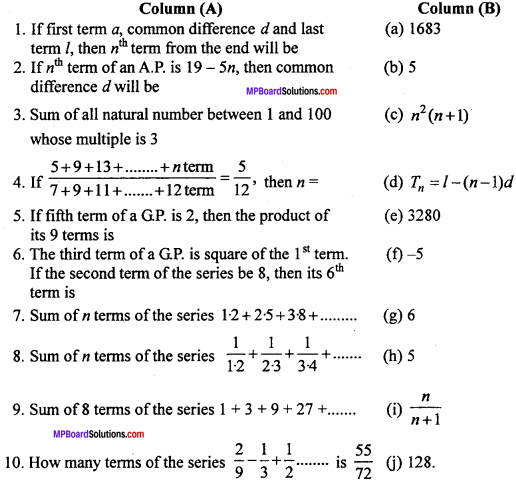1. (d)
2. (f)
3. (a)
4. (g)
5. (b)
6. (j)
7. (c)
8. (i)
9. (e)
10. (h)

(C) Fill in the blanks :

1. – 7$$\sqrt { 3 }$$ will be term of the series 5$$\sqrt { 3 }$$, 3$$\sqrt { 3 }$$, $$\sqrt { 3 }$$, …………….
2. 116 is sum of the term of the series 25,22, 19 …………….
3. If sum of n terms of series is n2 + 4n, then 15th term of the series is …………….
4. 0 will be the term of the series 27, 24,21, 18 …………….
5. 729 will be the term of the series – $$\frac {1}{27}$$, $$\frac {1}{9}$$, – $$\frac {1}{3}$$ …………….
6. The first term of GP. is a, common ratio r < 1 and its last term l, then its sum will be …………….
7. The ratio of the sum of first three term to the sum of first six term is 125 : 152, then common ratio will be …………….
8. First term 16 and fifth term $$\frac {1}{16}$$, then its 4th term will be …………….
9. Sum of n terms of the series x + 2x2 + 4x3 + 8x4 + ……………. will be …………….
10. If a = 2, d = 2 and n = 50, then the last term of the series will be …………….

1. 7
2. 12
3. 33
4. 10
5. 10
6. $$\frac {a – rl}{1 – r}$$
7. $$\frac {3}{5}$$
8. $$\frac {1}{4}$$
9. $$\frac { 1-({ 2x }^{ n })x }{ 1 – 2x }$$
10. 100

(D) Write true / false :

1. 1, 3, 5, 8, ………….. are inA.P.
2. nth term of a G.P. is a + (n – 1 )d.
3. If 2x, x + 5 and x + 11 are in A.P., then value of x will be – 1.
4. Arithmetic mean of a and b is $$\sqrt { ab }$$
5. Sum of 9 terms of a sequence 24 + 20 + 16 + will be.
6. Four consicutive term of G.P. are $$\frac { a }{ { r }^{ 3 } }$$ $$\frac {a}{r}$$, ar, ar3.

1. False
2. False
3. True
4. False
5. True
6. True.

(E) Write answer in one word / sentence :

1. If a, b, care inA.P., then find the value of ab + ac?
2. Arithmetic mean of (a + b)2and (a – b)2 will be?
3. Sum of n arithmetic mean between x and 3x will be.
4. Find the sum of infinite terms of series : 91/3.91/3.91/27 ………….. up to ∞.
5. If a, b, c are in GP., then find the value $$\frac {1}{b}$$ + $$\frac {1}{a – b}$$ –$$\frac {1}{b – c}$$

1. 2b2
2. a2 + b2
3. 3nx
4. 3
5. 0

Sequences and Series Short Answer Type Questions

Question 1.
Which term of the sequence 27,24,21,18, …………. is zero? (NCERT)
Solution:
Here a = 27 and d= T2 – T1 = 24 – 27 = – 3.
Let the nth term of series be 0.
∴ Tn = a + (n – 1)d
⇒ 0 = 27 + (n – 1)(- 3)
⇒ 3n – 3 = 27
⇒ 3n = 30
n = 10 or 10th term.

Question 2.
The last term of the series 8,4,0, ……………. is – 24. Find the total number of terms. (NCERT)
Solution:
Given series 8,4, 0, … (1)
First term = a = 8 Common difference d = 4 – 8 = -4
d = 0 – 4 = – 4
∵ The common difference is same the series is in A.P.
last term l = – 24,
l = a + (n – 1)d,
= – 24 = 8 + (n – 1)(-4)
⇒ – 24 – 8 = (n – 1)(-4)
⇒ – 32 = (n – 1)(- 4)
⇒ (n – 1) = $$\frac {32}{4}$$
⇒ n – 1 = 8
⇒ n = 8 + 1
⇒ n = 9
∴ Number of terms n = 9

Question 3.
Seven times the 7th term of a series is equal to eleven times of its 11th term. Find the 18th term of the series. (NCERT)
Solution:
Let first term = a and common difference = d of A.P.
∴ 7th term = a + 6d and 11th term = a + 10 d.
∴ According to question,
7(a + 6d) = 11 + (a + 10d)
⇒ 7a + 42d = 11a + 110d
⇒ 7a – 11a = 110d – 42d
⇒ – 4a = 68 d
⇒ a = – 17d
Hence 18th term = a + 17d
= – 17d + 17d [∵ a = – 17d]
= 0.

Question 4.
Prove that the sum of (m + n)th term and (m – n)th terms of an A.P. is twice of its mth term.
Solution:
Let the first term = a and common difference = d.
Tn = a+(n – 1)d
Tm + n= a + (m + n – 1)d … (1)
Tm – n = a + (m – n – 1)d … (2)
Tm = a + (m – 1)d … (3)
Tm+n + Tm-n = a + (m + n – 1)d + a(m – n – 1)d
= 2a + (m + n – 1 + m – n – 1)d
= 2a + (2m – 2)d
= 2a + 2(m – 1)d
= 2[a+(m – 1)d]
Tm+n + Tm-n = 2Tm.Question 5.
Insert three Arithmetic means between 3 and 19. (NCERT)
Solution:
Let three Arithmetic mean be A1, A2, A3,
then 3, A1, A2, A3, 19 are in A.P.
∴ 3 = a, 19 = T5, let common difference = d
T5 = a + 4d
T5 = a + 4d
⇒ 19 = 3 + 4d
⇒ 16 = 4d
⇒ d = 4
Hence A1 = 3 + 4 = 7, A2 = 7 + 4 = 11, A3 = 11 + 4 = 15.

Question 6.
If – 8, A1, A2 are in Arithmetic progression (A.P.), then find the value of A1, A2. (NCERT)
Solution:
– 8, A1, A2, 9 are in A.P.
∴ a = – 8, T4 = 9, let common difference = d
T4 = a + 3d
⇒ 9 = – 8 + 3 d
⇒ 3d = 11
⇒ d = $$\frac {17}{3}$$

Instruction :
Write the first five terms of each of the sequence and obtain the corresponding series.

Question 7.
(a) a1 = 3, an = 3an – 1 + 2, where n > 1. (NCERT)
Solution:
Given : a1 = 3,
a2 = 3an – 1 + 2, where n > 1
a2 = 3a2 – 1 + 2
⇒ a2 = 3a1 + 2
⇒ a2 = 3 x 3 + 2 = 9 + 2 = 11
a3 = 3a1 + 2
= 3a2 + 2
⇒ a3 = 3(11)+ 2 = 33+ 2 = 35
a4 = 3a4 – 1 + 2
= 3a3 + 2
⇒ a4 = 3(35) + 2 = 105 + 2 = 107
a5 = 3a5 – 1 + 2
= 3a4 + 2
= 3(107) + 2 = 321 + 2 = 323
Hence series is 3, 11, 35, 107, 323.

Question 7.
(b) a1 = -1, an = $$\frac { { a }_{ n-1 } }{ n }$$ where n ≥ 2.
Solution:Question 8.
A person pays first instalment of Rs. 100 towards his loan. If he increases his instalment every month by Rs. 5, then what will be his 30th instalment.
Solution:
Given : a = Rs. 100, d= Rs. 5, n = 30,
Tn = a + (n – 1)d
Amount of 30th instalment
J30 = 100 + (30 – 1) x 5
= 100 + 29 x 5 = 100 + 145
= Rs. 245.
Hence the 30th instalment = Rs. 245.

Question 9.
Which term of the sequence $$\sqrt { 3 }$$, 3, 3$$\sqrt { 3 }$$ ……. is 729?
Solution: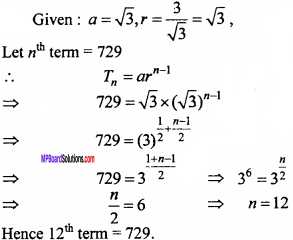Question 10.
How many terms are required in GP. 3,32,33 …………, so that their sum would be 120? (NCERT)
Solution:
Given : a = 3, r = $$\frac {9}{3}$$ = 3, Sn = 120,
Sn = $$\frac { a({ r }^{ n }-1) }{ r-1 }$$
⇒ $$\frac { 3({ 3 }^{ n }-1) }{ 3-1 }$$ = 120
⇒ 3n – 1 = $$\frac {120 × 2}{3}$$
⇒ 3n – 1 = 80
⇒ 3n = 81
⇒ 3n = 34
⇒ n = 4

Question 11.
Show that ratio between sum of n terms and sum of (n +1)th term to (2n)th terms in a G.P. is $$\frac { 1 }{ { r }^{ n } }$$
Solution:
Let 1st term of G.P. = a and common ratio = r
∴ n terms of GP. is a, ar, ar2, ………….. , arn – 1
Let the sum to n terms be S1
S1 = $$\frac { a({ r }^{ n }-1) }{ r-1 }$$
GP. from (n + 1)th term to (2n)th term
arn, arn + 1, …………. , ar2n – 1
Let the sum of this G.P. up to n terms be S2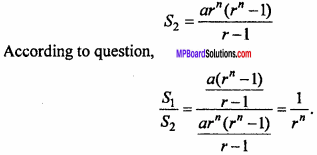Question 12.
If A.M. and GM. of roots of a quadratic equation are 8 and 5 respectively, then find the quadratic equation. (NCERT)
Solution:
Let the roots of equation be α and β
A.M. of roots = $$\frac {α + β}{2}$$ = 8
⇒ α +β = 16
G.M. of roots = $$\sqrt {αβ }$$ = 5
⇒ αβ = 25
If α, β are the roots of equation
then, x2 – (α + β)x + αβ = 0
⇒ x2 -16x + 25 = 0.Question 13.
If the first and nth term of GP. are a and b respectively and if p is the product of n terms, then prove that
p2 = (ab)n. (NCERT)
Solution:
Let the common ratio of G.P. = r
Given: arn – 1 = b, …(1)
then a.ar.ar2……. arn – 1 = p
⇒ anr1+2+3+……+(n – 1) = p
⇒ anr$$\frac {1}{2}$$(n – 1)n = p
⇒ a2n.r(n – 1)n = p2
⇒ p2 = (a2rn – 1n
⇒ p2 = (a.arn – 1)n
⇒ p2 = (ab)n, [fromeqn. (1)]

Question 14.
If the 4th term of a GP. is square of its second term and the 1st term is -3, then find the 7th term. (NCERT)
Solution:
Let a and r be the 1st term and common ratio of GP.
∴ Tn = arn-1
T4 = ar4-1 = ar3 … (1)
T2 = ar2-1 = ar … (2)
Given : T4 = (T2)2
⇒ ar3 = (ar)2
⇒ ar3 = a2r2
⇒ a = r = – 3 (given, a = -3)
T7 = ar7-1 = ar6
= (-3)(-3)6 = (-3)7
∴ T7 = – 2187
Hence 7th term of G.P. = – 2187.

Question 15.
Find the value of $$\sum _{ k=1 }^{ 11 }{ (2+{ 3 }^{ k }) }$$.
Solution: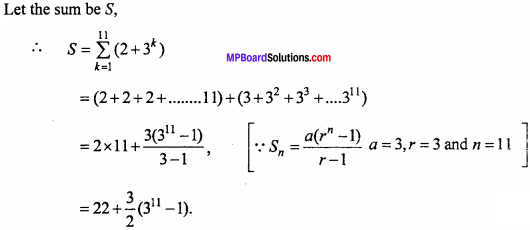Sequences and Series Long Answer Type Questions

Question 1.
If m times of the mth term of an A.P. is equal to n times the nth term, then prove that (m + n)th term of the series is zero.
Solution:
Let the 1st term = a and common difference = d.
then tm = a + (m – 1)d
tn = a + (n – 1)d
Given: m[a + (n – 1)d] = n[a + (n – 1)d]
⇒ ma + m(m – 1 )d = na + n(n – 1 )d
⇒ (m2 – m)d + (m – n)a = (n2 – n)d
⇒ (m2 – m – n2 + n) d + (m – n)a = 0
⇒ [m2 – n2 – (m – n)] d + (m – n)a = 0
⇒ [(m – n)(m + n) – (m – n)] d+(m – n)a = 0
⇒ (m – n)[(m + n) – 1] d + (m – n)a = 0
⇒ a + (m + n – 1 )d = 0
⇒ tm+n=0.

Question 2.
If the 6th term of A.P. is 12 and 9th term is 27, then find its rth term.
Solution:
Let the 1st term = a and common difference = d.
Given : t6 = 12
⇒ a + 5d = 12 … (1)
t9 = 27
⇒ a + 8d = 27 … (2)Question 3.
If pth term of an A.P. is $$\frac {1}{q}$$ and qth term is $$\frac {1}{p}$$, then prove that (pq)th
Solution: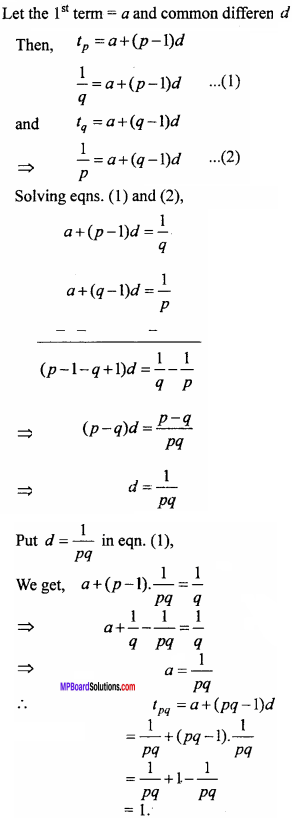Question 4.
If pth term of an A.P. is $$\frac {1}{q}$$ and qth term is $$\frac {1}{p}$$, then prove that (pq)th terms is $$\frac {1}{2}$$ (pq + 1), where p ≠ q.
Solution:Question 5.
The sum of the series 25, 22, 19, ………….. of A.P. is 116, then find its last term. (NCERT)
Solution:
Given : a = 25, d = 22 – 25 = – 3, Sn = 116
Sn = $$\frac {n}{2}$$ [2a + (n – 1)d]
⇒ 116 = $$\frac {n}{2}$$[2 x 25 + (n -1) x (-3)]
⇒ 232 = n[50 – 3n + 3]
⇒ 232 = n[53 – 3n]
⇒ 232 = 53n – 3n2
⇒ 3n2 – 53n +232 = 0
⇒ 3n2 – 24n – 29n + 232 = 0
⇒ 3n(n – 8) – 29(n – 8) = 0
⇒ (n – 8)(3n – 29) = 0
n = 8, or n = $$\frac {29}{3}$$, (which is impossible)
∴ Last term l = a + (n – 1 )d
= 25 + (8 – 1) x (- 3)
⇒ l = 25 – 21 = 4.Question 6.
If the A.M. of a and b is $$\frac { { a }^{ n }+{ b }^{ n } }{ { a }^{ n – 1 }+{ b }^{ n – 1 } }$$, then find the value of it. (NCERT)
Solution:
A.M. of a and b = $$\frac {a+b}{2}$$
According to question,
$$\frac { { a }^{ n }+{ b }^{ n } }{ { a }^{ n – 1 }+{ b }^{ n – 1 } }$$ = $$\frac {a+b}{2}$$
⇒ 2an + 2bn = (a + b) (an – 1 + bn – 1)
⇒ 2an +2bn = an + bn + abn – 1 + ban – 1
⇒ an + bn = abn – 1 + ban – 1
⇒ an – ban – 1 = abn – 1 – bn
⇒ an – 1(a – b) = bn – 1(a – b)
⇒ an – 1 = bn – 1
⇒ $$\frac {a}{b}$$n – 1 = $$\frac {a}{b}$$0
⇒ n – 1 = 0
⇒ n = 1.

Question 7.
Between 1 and 31, m numbers have been inserted in such a way that the resulting sequence is an A.P. and the ratio of 7th and (m – 1)th mean is 5 : 9 then, find the value of m.
Solution:
Let A1, A2, A3, ……… ,Am are m A.M. respectively inserted between 1 and 31.
Then 1, A1, A2, A3, ……… Am 31 are in A.P. Here, 1st term = 1 and (m + 2)th term = 31 and let the common difference of sequence be d.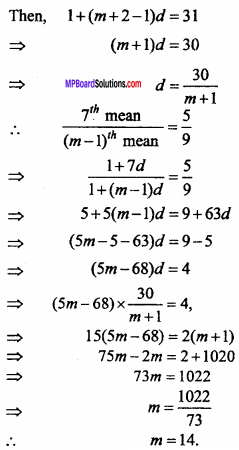Question 8.
Show that the sum of (m + n)th and (m – n)th term of an A.P. is equal to twice the mth term. (NCERT)
Solution:
Let the first term = a and common difference = d.
Tn = a + (n – 1)d
Tm + n =a +(m + n – 1)d … (1)
Tm – n = a + (m – n – 1)d … (2)
Tm = a + (m – 1)d … (3)
Tm + n + Tm – n = a + (m + n – 1)d + a + (m – n – 1)d
= 2a + (m + n – 1 + m – n – 1)d
= 2a + (2m – 2 )d
= 2a + 2 (m – 1)d
= 2[a + (m – 1)d]
∴ Tm + n + Tm – n = 2Tm [From equation (3)]Question 9.
If the sum of three numbers in A.P. is 24 and their product is 440, then find the numbers? (NCERT)
Solution:
Let the three numbers of A.P. are a – d, a, a + d.
Given : a – d + a + a + d = 24
3a = 24
⇒ a = 8
and (a – d) × a × (a + d) = 440
a(a2 – d2) = 440
⇒ 8(64 – d2) = 440
⇒ 64 – d2 = $$\frac {440}{8}$$
⇒ 64 – d2 = 55
⇒ d2 = 64 – 55
⇒ d2 = 9
⇒ d = ± 3
When a = 8 and d = 3
Then, a – d = 8 – 3 = 5, a = 8, a + d= 8 + 3 = 11
When a = 8 and d = – 3
Then, a – d = 8 + 3 = 11, a = 8, a + d = 8 – 3 = 5
Hence required numbers are 5, 8, 11 or 11, 8, 5.

Question 10.
If the sum of n, 2n and 3n terms in A.P. are S1 S2 and S3, then show that S3 = 3(S2 – S1).
Solution:Question 11.
Find the sum of all natural numbers lying between 200 and 400, which are divisible by 7. (NCERT)
Solution:
Numbers divisible by 7 are 203, 210, 217, …………. , 399
Here a = 203, d = 210 – 203 = 7, l = 399
l = a + (n – 1)d
399 = 203 + (n – 1) x 7
⇒ 399 – 203 = (n – 1) x 7
⇒ (n – 1)7 = 196
⇒ (n – 1) = $$\frac {196}{7}$$
⇒ (n – 1) = 28
∴ n = 29.
Hence required sum, S29 = $$\frac {n}{2}$$[a+l]
⇒ S29 = $$\frac {29}{2}$$[203 + 399]
⇒ S29 = $$\frac {29}{2}$$ = $$\frac {17458}{2}$$ = 8729.

Question 12.
If the 5th, 8th and 11th terms of a GP. are p, q and s respectively, then show
that q2 = ps. (NCERT)
Solution:
Let the 1st term of GP. = a and common ratio = r.
Then, Tn = arn – 1
T5 = ar5 – 1 =p
⇒ ar4 = P … (1)
T8 = ar8-1 = q
⇒ ar7 = q … (2)
T11 = ar11 – 1 = s
⇒ ar10 = s
ps = ar4.ar10, [from equation (1) And (3)]
⇒ ps = a2r14
⇒ ps = (ar7)2
⇒ ps = q2, [from equation (2)]
∴ q2 = ps.

Question 13.
If the first term of G.P. a = 729 and 7th term is 64, then find S7. (NCERT)
Solution: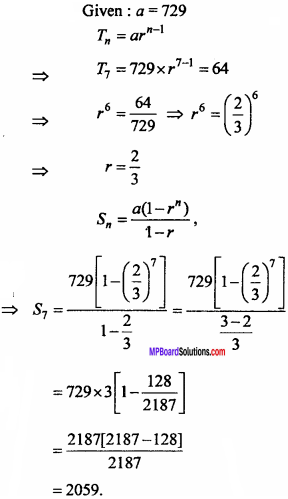Question 14.
If the 4th terms of a GP is square of its 2nd term and first term is – 3, then find its 7th (NCERT)
Solution:
Let the 1st term of GP. = a and common ratio = r.
Then, Tn = arn – 1
T4 = ar4 – 1 = ar3 … (1)
T2 = ar2-1 = ar … (2)
Given : T4 = T22
ar3 = (ar)2
ar3 = a2r2
⇒ ar3 = a2r2
⇒ a = r = – 3 (given a = – 3)
T7 = ar7 – 1 = ar6
= (-3)(-3)6 = (-3)7
∴ T7 = – 2187
Hence, 7thterm of G. P. = – 2187.

Question 15.
Find the sum of the sequence 8,88,888,8888 ……….. up to n terms. (NCERT)
Solution:
Let the sum of the n terms be SQuestion 16.
Find the sunt of the numbers 7,77,777, 7777 up to n terms. (NCERT)
Solution:
Let the sum of n terms is S.Question 17.
If the A.M. and GM. between two positive numbers a and b are 10 and 8 respectively, then find the numbers. (NCERT)
Solution:
A.M. A = $$\frac {a+b}{2}$$
a + b = 20 … (1)
G.M. G = $$\sqrt {ab}$$
ab = 64 … (2)
(a – b)2 = (a + b)2 – 4ab
= (20)2 – 4 × 64, [From equation (2)]
= 400 – 256
(a – b)2 = 144 = (12)2 … (3)Put a = 16 in equation (1), we get,
16 + b = 20
∴ b = 4
Numbers are 16 and 4.
When a – b = – 12, then
a + b = 20
a – b = – 12
⇒ a = 4
Put a = 4 in equation (1), we get,
4 + 6 = 20
∴ b = 16
Numbers are 4 and 16.
Hence numbers a and b are 4, 16 or 16,4.Question 18.
The sum of two numbers is 6 times their geometric mean, show that the . numbers are in the ratio (3 + 2$$\sqrt {2}$$) ; (3 – 2$$\sqrt {2}$$). (NCERT)
Solution:
Let the numbers are a and 6.
Given: a + b = 6 $$\sqrt {ab}$$
$$\frac { a+b }{ 2\sqrt { ab } }$$ = $$\frac {3}{1}$$
⇒ a + b = 3K …. (1)
and 2 $$\sqrt {ab}$$ = K ⇒ 4ab = K2
(a – b)2 = (a + b)2 – 4ab
= (3K)2 – (K)2
= 9K2 – K2 = 8K2
⇒ a – b = 2$$\sqrt {2}$$K …. (2)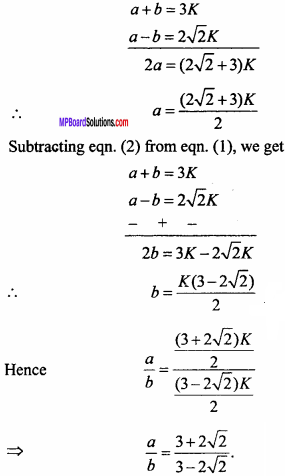Sequences and Series Very Long Answer Type Questions

Question 1.
If the ratio of the sum of n terms of two A.P. is 5n + 4 : 9n + 6, then find the ratio of their 18th term.
Solution:
Let the two A.P. are :
a, a + d, a + 2d, ………….
and A, A + D, A +2D …………..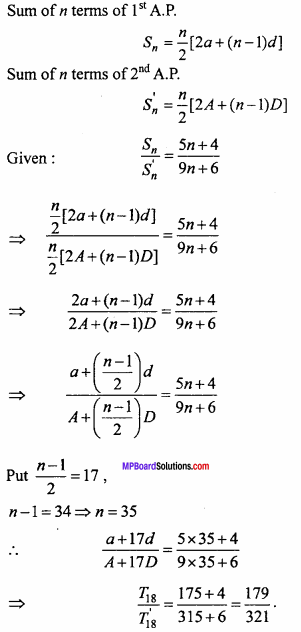Question 2.
The ratio of the sum of m and n terms of an A.P. is m2 : n2. Show that the ratio of and u* term is (2m – 1) : (2n – 1). (NCERT)
Solution:
Let the A.P. are a, a + d, a + 2d, ……………
∴ mth term of A.P. Tm = a + (m – 1)d
nth term of A.P. Tn= a + (n – 1)dQuestion 3.
If the sum of first three terms of a G.P. is $$\frac {39}{10}$$ and their product is 1, then find the common ratio and the terms. (NCERT)
Solution:
Let the three terms of G.P. are $$\frac {a}{r}$$, a, ar.
Given: $$\frac {a}{r}$$ × a × ar = 1
⇒ a3 = 1 ⇒ a = 1
and $$\frac {a}{r}$$ + a + ar = $$\frac {39}{10}$$
⇒ a($$\frac {a}{r}$$ + r + 1) = $$\frac {39}{10}$$
⇒ 1 x $$\frac { 1+{ r }^{ 2 }+r }{ r }$$ = $$\frac {39}{10}$$
⇒ 10r2 + 10r + 10 = 39r
⇒ 10r2 – 29r + 10 = 0
⇒ 10r2 – 25r – 4r + 10 = 0
⇒ 5r(2r – 5) – 2(2r – 5) = 0
⇒ (5r – 2)(2r – 5) = 0
∴ r = $$\frac {2}{5}$$ and r = $$\frac {5}{2}$$
When a = 1 and r = $$\frac {2}{5}$$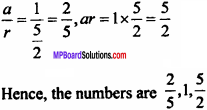Question 4.
Find four numbers forming a G.P. in which the third term is greater than the lint term by 9 and the second term is greater than 4th term by 18. (NCERT)
Solution:
Let the four terms of G.P. be a, ar, ar2,ar3.
Given: T3 = T1 + 9
⇒ T3 = T1 + 9
⇒ T3 = a + 9
⇒ ar2 = a + 9 …. (1)
According to question,
T2 = T4 + 18
⇒ ar = a3 + 18
⇒ ar – ar3 = 18 …. (2)Put r = – 2 in equation (1), we get
a(- 2)2 – a = 9
⇒ 4a – a = 9
⇒ 3a = 9
⇒ a = 3
∴ Numbers are 3, 3(- 2), 3(- 2)2, 3(-2)3, ……………..
3, – 6, 12, – 24, ………………

Question 5.
If S be the sum of it terms of a GP., P be the product and R be the sum of reciprocal of n terms, then prove that
P2Rn = Sn. (NCERT)
Solution:
Let n terms of GP. be a, ar, ar2, …………… arn – 1.
According to the question,Question 6.
If x = 1 + a + a2 + ………….∞ ($$\left| a \right|$$<1)
y = 1 + b + b2 + …………….∞ ($$\left| b \right|$$<1)
then prove that
1 + ab + a2b2 + …………….∞ = $$\frac {xy}{x + y – 1}$$
Solution:Question 7.
The sum of infinite terms of a Geometric Progression is 15 and sum of the square of its terms is 45. Find the Geometric Progression. (NCERT)
Solution:
Let a be the first term and r be the common ratio.
∵ $$\left| r \right|$$<1,
Then, $$\frac {a}{1 – r}$$ = 1.5 …. (1)
Squaring the terms of GP. the new G.P. is
a2, a2 r2, a2 r4, a2 r6, ……….
Sum of infinity of G.P. = $$\frac { { a }^{ 2 } }{ { 1 – r }^{ 2 } }$$.
$$\frac { { a }^{ 2 } }{ { 1 – r }^{ 2 } }$$ = 45, (given) … (2)
Squaring both sides of equation (1) and the result is divided by equation (2),
$$\frac { { a }^{ 2 } }{ { 1 – r }^{ 2 } }$$2 × $$\frac { 1-{ r }^{ 2 } }{ { a }^{ 2 } }$$ = $$\frac {15×15}{45}$$
⇒ $$\frac {1 + r}{1 – r}$$ = 5
⇒ 1 + r = 5 – 5r
⇒ 6r = 4
⇒ r = $$\frac {2}{3}$$
Put r =$$\frac {2}{3}$$ in equation (1), we getHence required progression is 5, 5 × $$\frac {2}{3}$$, 5 × ($$\frac {2}{3}$$)2, …………….
Hence 5, $$\frac {10}{3}$$, $$\frac {20}{9}$$, ………………

Question 8.
A farmer buys a used tractor of Rs. 12,000. He pays Rs. 6,000 cash and agrees to pay the balance in annual instalment of Rs. 500 plus 12% interest on theunpaid amount How much the tractor cost him?
Solution:
Cost of tractor = Rs. 12, 000, down payment = Rs. 6,000
Balance amount = 12,000 – 6,000 = Rs. 6,000Actual cost of tractor = 12,000 + 4,680 = Rs. 16,680. Ans.

Question 9.
Shamshad Ali buys a scooter for Rs. 22,000. He pays Rs. 4,000 cash and agrees to pay the balance in annual instalment of Rs. 1,000 plus 10% interest on the unpaid amount How much will scooter cost him? (NCERT)
Solution:
Cost of scooter = Rs. 22,000, Cash down payment = Rs. 4,000.
Remaining amount = 22,000 – 4,000 = Rs. 18,000Actual cost = 22,000 + 17,100 = Rs. 39,100.
Total amount paid for scooter = Rs. 39,100.

Question 10.
A person writes a letter to four of his friends. He asks each one of them, to copy the letter and mail to four different persons with instructions that they move the chain similarly. Assuming that the chain is not broken and that is costs 50 paisa to mail one letter. Find the amount on postage when 8th set of letter is mailed. (NCERT)
Solution:
First person sends 4 letters.
2nd step, he sends 4 x 4= 16 letters
3rd step, he sends 4 x 4 x 4 = 64 letters
Hence 4, 16, 64, 256, …………… is a Geometric series.
Here a = 4, r = $$\frac {16}{4}$$ = 4
Sn = $$\frac { { a(r }^{ n } – 1) }{ r – 1 }$$ [∵r>1]
∴ Total number of letters till 8th set = S8 = $$\frac { { 4(r }^{ 4 } – 1) }{ 4 – 1 }$$
= $$\frac {4}{3}$$ (65536 – 1) = $$\frac {4}{3}$$ x 65535
Cost for one letter = Rs. 0.50
∴ Hence total cost = $$\frac {4}{3}$$ x 65535 x 0.50 = Rs. 43690.Question 11.
150 workers were engaged to finish a job in a certain number of days, 4 workers dropped out on second day, 4 more workers dropped out on third day and so on. It took 8 more days to finish the work. Find the number of days in which the work was completed. (NCERT)
Solution:
150, 146, 142, 138, …………, it is a Geometric series.
Let the number of days required complete the work be n.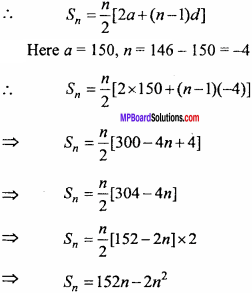If the workers not dropped then the work would have complited in (n – 8) days with 150 workers working on each day.
Hence the total workers worked for n days = 150 (n – 8)
= 150n – 1200 ….. (2)
From equation (1) and (2),
150n – 1200 = 152n – 2n2
2n2 + 150n – 152n – 1200 = 0
2n2 – 2n – 1200 = 0
n2 – n – 600 =0
n2 – 25n + 24n – 600 = 0
n(n – 25) + 24(n – 25) = 0
(n – 25)(n + 24) = 0
n =25 and n = – 24 (not possible)
∴ Work is completed in 25 days.